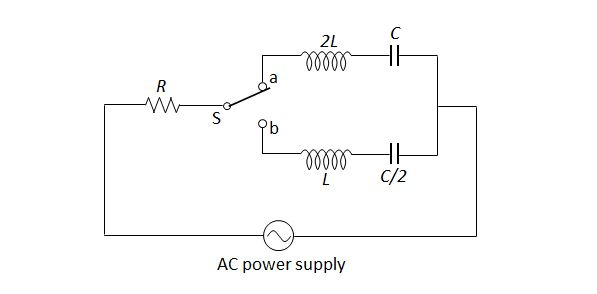# RLC Circuit 3The above is an electric circuit, where a resistor, $2$ inductors and $2$ capacitors are connected to an AC power supply. The maximum voltage and the frequency of the AC power supply is $V_0$ and $\displaystyle \frac{1}{2\pi\sqrt{LC}},$ respectively. If the switch S is connected to $a$, then the maximum value of the current intensity flowing in the circuit is $I_1,$ and the maximum value of the voltage across the resistor is $V_1.$ Which of the following statements is correct when the switch S is connected to $b?$

a) The impedance of the circuit is $R.$

b) The maximum value of the current intensity flowing in the circuit is $I_1.$

c) The maximum value of the voltage across the resistor is $V_0.$

×

Problem Loading...

Note Loading...

Set Loading...